Although it may seem obvious, knowing how to correctly calculate the exact number of tiles you will need is very important as the number of tiles that are going to be cut and installed is not the same as the amount you need to buy.

To start, you need to calculate the area of the room you’re tiling. For this, you will need a basic knowledge of geometry, some small but key details of each job, the material you’re tiling with and a pencil and paper or calculator to hand.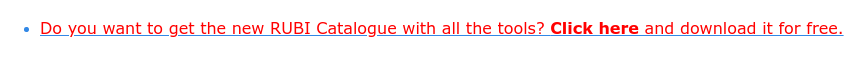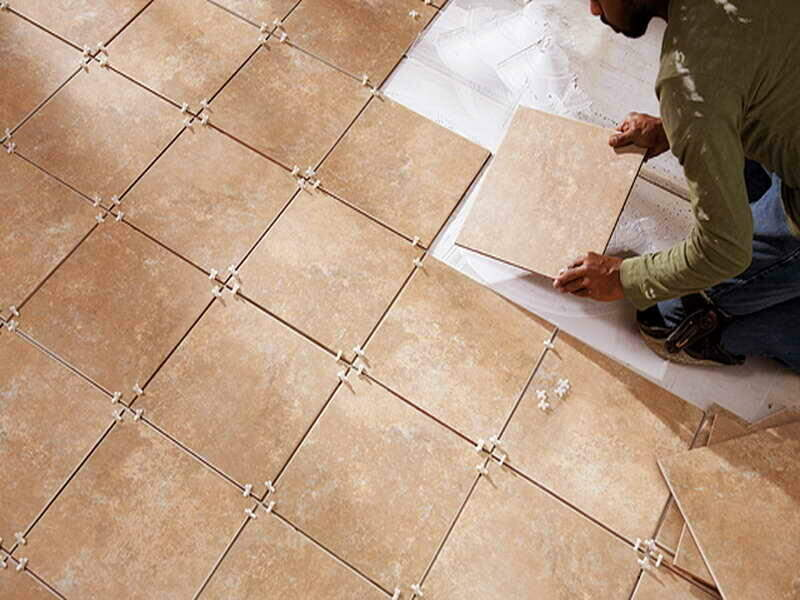## Calculating the area of the room

The first thing we must do is identify and measure the surface of the work area .

That is, the surface where the ceramic tile will be placed. Of course, if we talk about several walls or different floors, in the end, we will only have to add them all. That, of course, applies if we are going to use the same tile model for all of them.

First thing to do is measure the surface of your work area. To do this, you simply need to measure the Height x Width.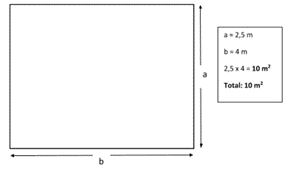If you are planning to use different sizes in the same installation, we will have to calculate the square meter for each tile model, independently.

## Calculation for multiple sized tiles

In order to calculate a surface that will be made up of more than one geometric shape you need to break-down the total area into simple forms. And also calculate each area separately to add them all in at the end.One of the most complex situations will be one when one or more of the sides of your tile is not perpendicular. In this case, to calculate the tiles per square meter, we will opt for a similar same procedure, but applying a triangular calculation.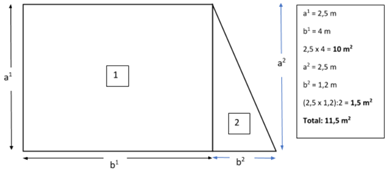## What do I do about the doors and windows?

As you are not going to be tiling the doors or window, you will need to remove them from your calculation process. To do this, you simply have to measure the surface of these elements and subtract them from the total.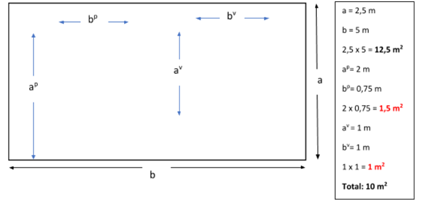## Time to go shopping

You have now finished calculating the number of tiles per square meter and you’re ready to head to the shops. But first, there’s a couple more details you must take into account

### 1. You need to add an “extra” amount

To begin with, you know the total work area you’re tiling. But you need to take into account that not all the tiles will be installed whole and, therefore, they will have to be cut. If we add to this, the possibility of a piece breaking or chipping in the attempt to cut the tile, you need to add an “extra” amount to cover these possible losses.

As a general rule, you should always add 10% to the total

However, if you’re planning to lay a herringbone pattern, we suggest adding 20%. You need to expect more wastage due to the pattern and design you will have to lay.

We almost have everything together!### 2. How many square meters you need to buy

The last thing you need to consider is how many square meters you need to buy. Not the number of tiles per square meter as it’s not common for tiles to be sold individually. Therefore, you will buy them by the box and look at what the box tells you the total surface it will cover.

It’s very rare you’ll find boxes that cover exactly 1 square meter. So, you will have to divide the total area (including the excess of waste you’ve added) by the area that the box covers.

And there you have it! The total number of boxes that you will need!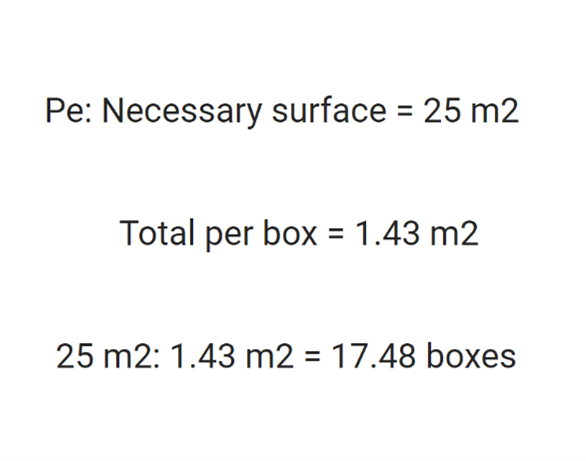As the boxes must be bought whole, you need to round up and we will have already completed our mission of calculating the number of tiles needed per square meter.

Remember, what we mentioned at the beginning: if you are going to combine different formats on floor and wall , then you will have to do the calculations separately.

As we’ve shown you, it’s not very complicated. Sometimes not taking into account these small details can generate a lot of problems and headaches down the line.